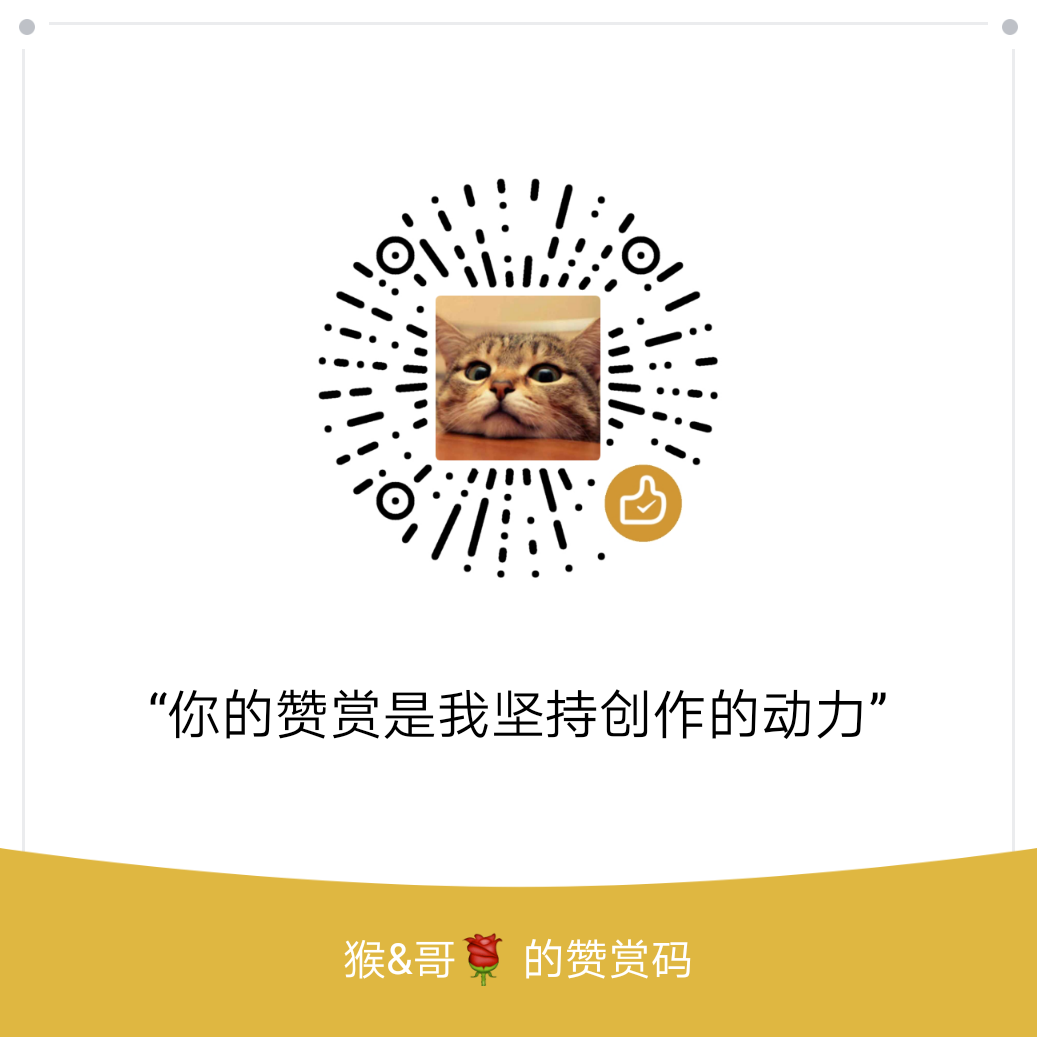• 欢迎访问极客猴，分享 Python 入门，网络爬虫，数据分析，赚钱思维相关的技术与思维`QQ群`
• 本站点文章首发于微信公众号【极客猴】，欢迎关注，第一时间接受文章推送~
• 如果您觉得本站非常有看点，那么赶紧使用 Ctrl+D 收藏极客猴吧

# 几个Python编程小技巧

3年前 (2018-01-23) 18次浏览

## 1.编码问题

``````# 数据库以 UTF-8 形式保存字符串，而获取到的字符串是 GB2312
str = getFromNetWork()      # 获取网络字符串，字符编码为 GB2312
str.encode('GB2312').decode('UTF-8')
print(str)``````

## 2.值交换

``````int a=10, b=20,temp;
temp = a;
a = b;
b = temp;``````

``````a = 10
b = 20
print('a = ', a, ' b = ', b)
b, a = a, b
print('a = ', a, ' b = ', b)

>>  a =  10  b =  20
>>  a =  20  b =  10``````

## 3.单例模式

Python 不仅是面向过程的编程语言，而且是面向对象的编程语言。在 Python 中，一个类被初始化，那么 `__new__()` 函数一定会先被调用，然后再调用`__init__()`。我们可以采用 hasattr() 函数来判断对象是否包含对应的属性，也就是判断类是否被初始化。

``````class Singleton(object):
def __new__(cls):
# 关键在于这，每一次实例化的时候，我们都只会返回这同一个instance对象
if not hasattr(cls, 'instance'):
cls.instance = super(Singleton, cls).__new__(cls)
return cls.instance

obj1 = Singleton()
obj2 = Singleton()
obj1.name = 'GeeMonkey'
print(obj1.name, obj2.name)
print(obj1 is obj2)

>>  GeeMonkey GeeMonkey
>>  True``````

[赞赏码]